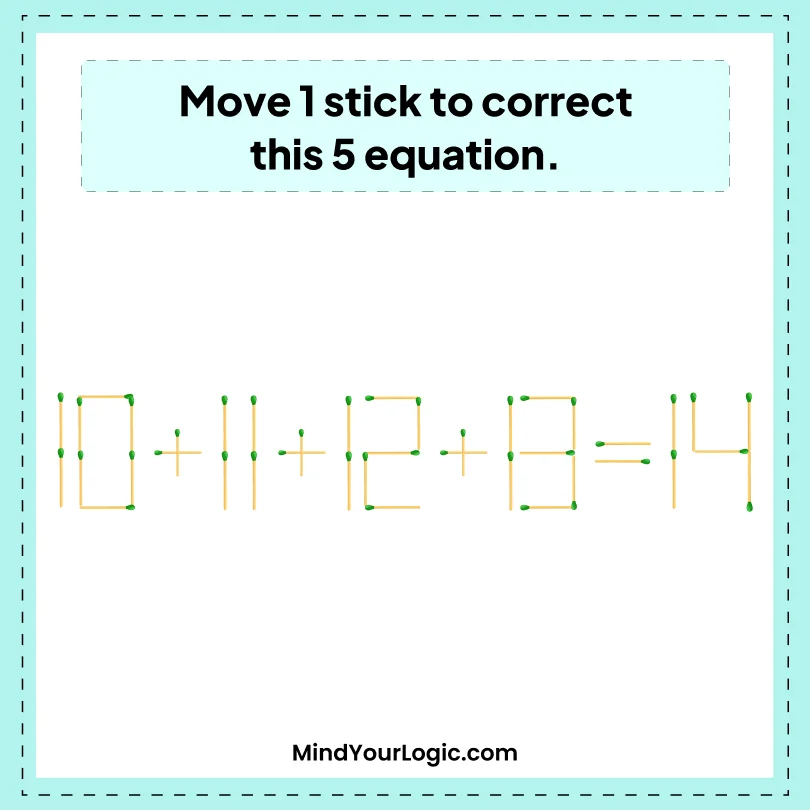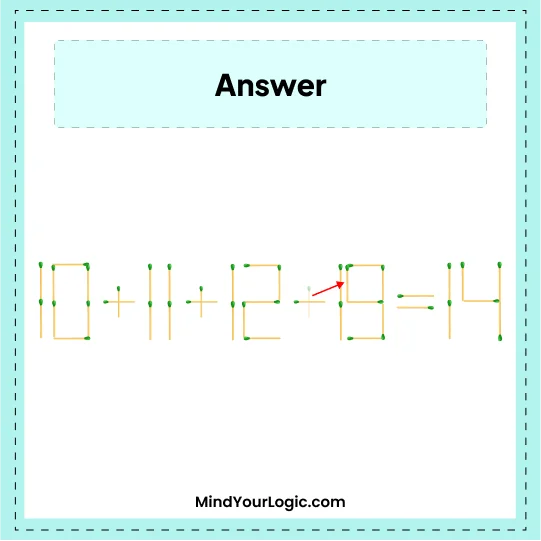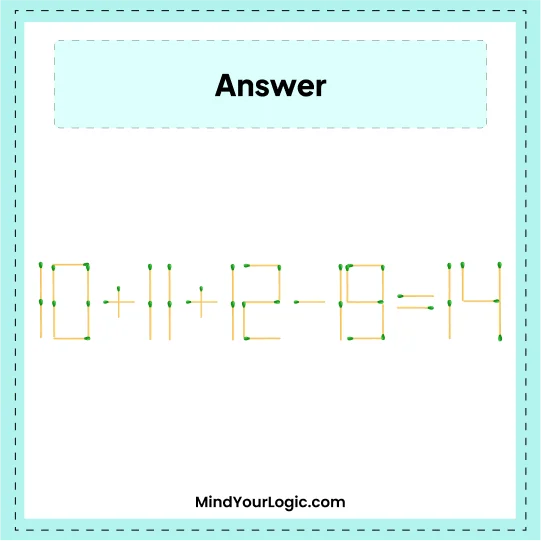# 10 + 11 + 12 + 13 = 14 - Matchstick Puzzles

###### 18.Matchstick Puzzles
`Move one stick to correct this five consecutive numbers wrong equation. `•

Explanation :

``` Move one stick from the 3rd + sign to 13, i.e. 3 becomes 9, the whole number becomes 19. So, the final equation we get is-
10  + 11  +  12  -  19 = 14.```